# ORIBATIDA Mode of Authenticated Encryption

Oribatida is a variant of the monkey-wrap design, as used before, e.g., in Ascon or NORX. Oribatida extends such previous designs by a ciphertext-block masking that boosts the security and ensures resilience against release of unverified plaintext material. We denote by (Ui, Vi) the outputs of and by (Xi, Yi) the inputs to the permutation. As in the classical sponge, Oribatida considers the state Si = (Ui||Vi) as a rate part Ui of r bits, where inputs are XORed to, and a capacity part Vi of c = n−r bits. Unlike the usual sponge, an s-bit part of the capacity is used to mask the subsequent ciphertext block.

## Features

1. Oribatida Is Lightweight. As a keyed permutation-based mode of operation, Oribatida has to store neither the state of the permutation (plus a small overhead) nor subkeys or tweaks. Authentication and encryption can be performed fully online and impose no need on buffering the input. For instantiations, we employ a lightweight family of permutations SimP that is very close to the block-cipher variants Simon-96-96 or Simon-128-128 and their respective key schedules. As a result, the instances of SimP possess a low state size of only 192 and 256 bits, respectively.

2. Oribatida Is Performant. For a security level of 128 bits, Oribatida provides a rate of 1/2 (two calls to a permutation for processing n-bit message material) for authentication and encryption even with a small permutation size of only n = 192 or n = 256 bits due to the way it masks ciphertext blocks.

3. Oribatida Alleviates Its Usage Across Different Platforms. Our proposed instantiations of Oribatida with the SimP family of permutations can be implemented with only the basic operations AND, rotations, and XOR. Since SimP avoids the use of S-boxes, implementations can split the state flexibly according to the target platforms’ needs.

4. Oribatida Is Based on Well-known Components. The design of Oribatida is based on the well-known duplex mode. Therefore, it founds on well-established results. Since SimP is very close to the design of Simon, it can profit from the existing cryptanalysis, and rely on its already well-understood permutation design.

5. Oribatida Is Secure. The design of Oribatida inherits the minimal security guarantees of the duplex mode. Moreover, Oribatida augments the usual sponge by a ciphertext masking that boosts the security. While this specification omits tedious proof details, all members of the Oribatida family are expected to provide 128-bit security for encryption and integrity.

6. Oribatida Is Robust under Release of Unverified Plaintexts. While authenticated encryption can be realized in an online manner, proper authenticated decryption must be offline. However, resource-constrained devices can hardly buffer long messages until the authentication tag is verified, which can lead to a complete loss of privacy and integrity. The ciphertext masking of Oribatida limits the security damage in such cases. In the case of accidental misuse, Oribatida provides integrity also in the case that plaintext material leaks from invalid ciphertexts.

## Mode Specification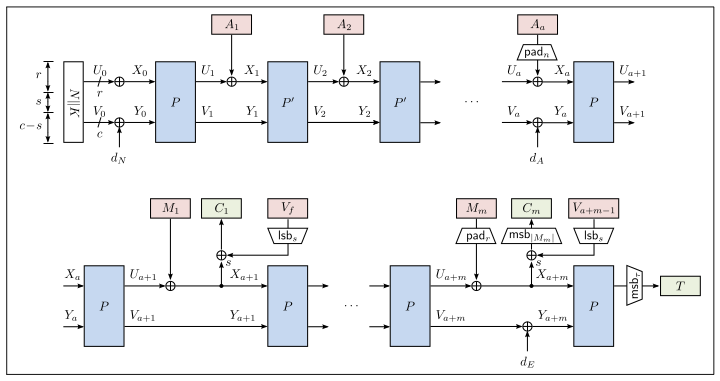Schematic illustration of the authentication of an a-block associated data A and the encryption of an m-block plaintext M with Oribatida, for m > 1. P and P' are permutations, K the secret key, N the nonce, C the resulting ciphertext, and T the resulting authentication tag.

### Initialization

Each variant of Oribatida uses a fixed size for nonces, whose length is chosen such that k+ν = n bits. The nonce N is concatenated with the key K to initialize the state: N||K: (U0, V0) ← (N||K) ⊕d dN. The domain dN is XORed to the least significant byte of the initial state. Then, the first state value S1 results from a call to the permutation: (U1||V1) ← P(U0||V0). Note that we store the value of V0 or V1 (aliased by Vf), depending on whether the associated data is empty or not, to mask the first block of ciphertext later.

### Processing Associated Data

After the initialization, the associated data A is padded with a 10∗-padding if |A| mod r ≠ 0 such that its length becomes the next highest multiple of r bits. Thereupon, the padded associated data A is split into r-bit blocks (A1, ... , Aa). Given the state Si = Ui||Vi, where |Ui| = r and |Vi| = c, Ai is XORed to the rate part of the state: Xi ← Ui⊕Ai, for 1 ≤ i < a. For all non-final blocks of A, the capacity part of the permutation output, Vi, is simply forwarded to the capacity part of the subsequent input to the permutation P': Yi ← Vi . The next state is computed by a call to the reduced permutation P' afterwards, for all indices 1 < i < a but the final a-th block of A: Si ← P'(Xi||Yi). When the final block Aa is processed, a domain dA that depends on the lengths of A and M is XORed to the least significant byte of the capacity.

### Encryption

After the associated data has been processed, the message M is encrypted. If the length of M is not a multiple of r bits, M is padded with a 10∗-padding such that its length after padding becomes the next highest multiple of r bits. Thereupon, M is split into r-bit blocks (M1, ... , Mm) after padding.
The blocks Mi are processed one after the other. Given the state value Si = Ui||Vi, where |Ui| = r and |Vi| = c, the current block Mi is XORed to the rate part Ua+i : Xa+i ← Mi⊕Ua+i. The capacity part is simply forwarded: Ya+i ← Va+i. Then, (Xa+i||Ya+i) is used as input to a call to P to derive the next state value Sa+i+1 ← P(Xa+i||Ya+i).
The ciphertext blocks Ci are computed from a sum of the current rate, the current plaintext block, and a (partial) earlier value from the capacity. The first ciphertext block is computed from C1 ← Xa+i⊕LSBs(Vf).
If it is the final block, then, C1 is computed from C1 ← MSBlE(Xa+i⊕LSBs(Vf)), where lE denotes the length of M before padding.
The further non-final ciphertext blocks Ci, 1 < i < m, are computed from Ci ← Xa+isLSBs(Va+i−1), for 1 < i < m. If m > 1, the final ciphertext block Cm is computed from Cm ← MSBlE mod r(Xa+m⊕LSBs(Va+m−1)).
For the final message block, a domain dE is XORed to the least significant byte of the capacity: Ya+m ← Va+mddE. Thereupon, P is called another time to derive Sa+m+1 ← P(Xa+m||Ya+m). Its rate part, truncated to τ bits if necessary, is released as the authentication tag: T ← MSBτ(Sa+m+1).

### Decryption

The decryption algorithm takes a tuple (K,N,A,C,T). Again, the initialization with K and N as well as the processing of the associated data A is performed in the same manner as for encryption. If |C| mod r ≠ 0, the decryption pads C with a 10∗-padding to the next multiple of r bits. In all cases, it splits C into r-bit blocks (C1, ... , Cm-1) plus a final block Cm. If m > 1, the plaintext block is computed as Xa+i ← Ci⊕LSBs(V), Mi ← (Ua+i⊕Xa+i), where V = Vf for i = 1 and V = Va+i−1 otherwise. The capacity is again simply forwarded to the next call of the permutation: Ya+i ← Va+i. The subsequent state is then computed by (Ua+i+1||Va+i+1) ← Sa+i+1 ← P(Xa+i||Ya+i).
For the final block m, the final plaintext block is computed from the padded ciphertext block Cm as Xa+m ← Cm⊕LSBs(V), Mm ← LSBx(Ua+m⊕Xa+m), where x ← lE mod r. For the final block, the domain dE is XORed to the least significant byte of the capacity: Ya+m ← Va+mddE. The would-be tag T' is derived by computing (T'||Z) ← P(Xa+m||Ya+m), and using only its most siginficant τ bits: T' ← MSBτ(T'||Z) as for the encryption. If T=T', the ciphertext is considered valid, and M = (M1|| ... ||Mm) is released as plaintext. Otherwise, the ciphertext is considered invalid, and ⊥ is given as output.

### Domain Separation

For the purpose of domain separation, Oribatida defines a set of domain constants dN, dA and dE. The domains are XORed with the least significant byte of the state at three stages. The value depends on the presence of A and M and whether their final blocks are absent, partial, or integral. This ensures that there exist no trivial collisions of inputs to P among blocks of A and M.
The constants are determined by the four control bits (t3, t2, t1, t0) that reflect inputs in the hardware API. The rationale behind them is the following:
• EOI: t3 is the end-of-input control bit. This bit is set to 1 iff the current data block is the final block of the input. For all other cases, t3 is set to 0.
• EOT: t2 is the end-of-type control bit. This bit is set to 1 iff the current data block is the final block of the same type, i.e., it is the last block of the message/associated data. Note that, if the associated data is empty, the nonce is treated as the final block of the associated data. So, t2 is set to 1. For all other cases, t2 is set to 0.
• Partial: t1 is the partial-control bit. It is set to 1 if the current data block is partial, i.e. if its size is less than the required block size. For all other data blocks, t1 is 0.
• Type: t0 is called the type-control bit. It identifies the type of the current data block. For the nonce and the processing of the final message block, t0 is set to 1. For all other cases, t0 is set to 0.
For example, if we are processing the nonce (which is always a complete r-bit block), where the associated data is empty, and the message is not empty, it holds that dN = (t3t2t1t0) = (0101).

## SimP-n-θ Family of Permutations

SimP-n-θ is a family of permutations from n bits to n bits. Each of them uses Simon(n/2)/(n/2) block cipher in θ rounds. The input to the permutation is divided into two halves, and they serve as the key and the input to the first round respectively. The key and the output of one round (each of size n/2 bits) serves as the input and the key of the next round respectively. In the end, the key and the output of the final round are concatenated and it serves as the output of the permutation. Each round deviates very little from the exact Simon block cipher algorithm. The detail of the SimP-n-θ family of permutations can be found here, and the detail of the Simon family of block ciphers can be found here.

## Recommended Instantiations

Oribatida-n-s is proposed in two versions, parametrized by the state size of the permutation n, and a mask size s. The following table lists the proposed parameter sets. We define a security parameter z = c+s which should be defined as 192 (the target for NIST security requirements). We briefly recall the parameters and the conditions satisfied by these parameters.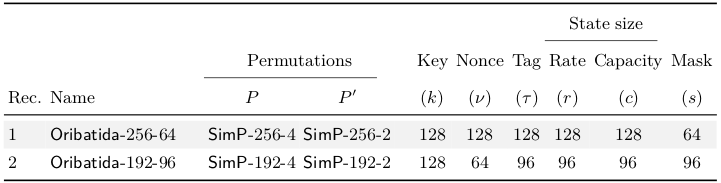Recommended schemes of Oribatida in the order of recommendation. The topmost is our primary recommendation. All integer values are given in bits. The state size is given by r + c. Rec. = Recommendation.
1. We always choose a key size of k = 128 bits.
2. n denotes the size of the permutation in bits, which is either 256 or 192.
3. The nonce length ν is chosen such that ν+k = n holds.
4. The capacity of the permutation is chosen as c = 192−s bits (by the security requirement mentioned above).
5. Thus, the mask size s is at most the capacity: s ≤ c.
6. Finally, the tag length is set to τ = r bits.
Oribatida-n-s employs two internal permutations P, P' ∈ Perm(Fn2), where P' is chosen as a round-reduced variant of P to process the associated data efficiently. The following members of the Oribatida-n-s family are proposed, based on instantiations from the SimP family of permutations:
• Our primary recommendation is Oribatida-256-64. For P, this variant uses SimP-256-4 with rs = 34 rounds per step and θ = 4 steps. Moreover, for P', it employs SimP-256-2 with rs = 34 rounds per step and θ = 2 steps.
• Our secondary recommendation is Oribatida-192-96. For P, this variant uses SimP-192-4 with rs = 26 and θ = 4 steps. For P', it employs SimP-192-2 with rs = 26 and θ = 2 steps.

## Security

The following table summarizes our security claims for our recommended variants of Oribatida.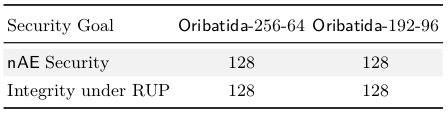Security claims in bits for our recommended schemes. RUP = release of unverified plaintext material.

## Cryptanalysis

The number of steps and rounds of SimP was chosen to resist known cryptanalysis techniques and tools. This section provides a rationale of our choices from the existing works.

### Existing Cryptanalysis on Simon

Various works considered analyzed the Simon family of block ciphers since its proposal.

Differential Cryptanalysis. Cryptanalysis that appeared early after the proposal of Simon followed heuristics for differential cryptanalysis: Abed et al. followed a heuristic branch-and-bound approach that yielded differentials for up to 30 round Simon-96. Biryukov et al. studied more efficient heuristics, but considered the small variants with state sizes up to 64 bits. Dinur et al. showed that distinguishers on Simon with k key words can be extended by at least k rounds. Interestingly, boomerangs seemed to be less a threat to Simon-like ciphers than pure differentials.
Kölbl et al. redirected the search on optimal characteristics. More recently, Liu et al. employed a variant of Matsui’s algorithm to find optimal differential characteristics. They found that characteristics with probability higher than 2−96 covered at most 27 rounds. Moreover, they found at best 31-round differentials with accumulated probability higher than 2−96, i.e., of probability 2−95.34. For Simon-128, they showed that optimal differential characteristics covered at most 37 rounds; furthermore, they found 41-round differentials with cumulative probability of 2−123.74.

Linear Cryptanalysis. Linear trails are similarly a threat as differential trails. Alizadeh et al. studied linear trails. They reported multi-linear distinguishers on all variants of Simon. For Simon-96-96, they proposed a multi-linear distinguisher on up to 31 rounds that could be extended by two rounds in a key-recovery attacks. Similarly, they reported a 37-round distinguisher for Simon-128-128 that could be extendable by two rounds. Chen and Wang published improved key-recovery attacks that employed dynamic key-guessing, i.e., adaptive guessing of key bits to reduce the complexity. Their attacks are the most effective ones for our considered variants to the best of our knowledge, covering up to 37 rounds of Simon-96-96 and up to 49 rounds of Simon-128-128 in theory.
Similar as for differentials, Liu et al. studied also optimal linear approximations with an algorithm adapted from Matsui. Liu et al. found that optimal linear approximations can reach at most 28 rounds for Simon-96, and at most 37 rounds for Simon-128. They further found linear hulls with potential of 2−93.8 for 31 rounds of Simon-96, and 2−123.15 for 41 rounds of Simon-128.

Integral, Impossible-differential, and Zero-correlation Distinguishers. Integral attacks cover at most 22 rounds for Simon-96-96 and 26 rounds of Simon-128-128. Zhang et al. found integrals on up to 21 and 25 rounds for Simon-96 and Simon-128. Their results were extended by one round each by Xiang et al., and later by Todo and Morii. The latter could show the absence of integrals for 25-round Simon-96. Their observation was confirmed by Kondo et al..
Shen et al. studied impossible-differential and zero-correlation distinguishers. The maximal number of rounds that such distinguishers can cover is given by at most twice the length of the maximal diffusion. Since this is given by 11 rounds for Simon-96 and 13 rounds for Simon-128-128 by Kölbl et al., such distinguishers can cover at most 22 and 26 rounds in the single-key setting.
For related keys, Kondo et al. searched for iterative key differences in Simon. This allowed them to extend previous results by four to 15 rounds. For Simon-96-96, the authors found iterative key differentials for up to 20 rounds. Though, it remains unclear if this yields an impossible differential; in the best case, such a 20-round distinguisher can be extended by 2+2+2 wrapping rounds: two more blank rounds where one of the key words is not used, plus two rounds where the key difference can be canceled by the state differences, plus two outermost rounds since the result of the non-linear function is independent of the key and therefore predictable in Simon. So, an impossible-differential distinguisher could cover up to 26 rounds. Note that this upper bound that has not been formulated to an attack on the here-considered versions by Kondo et al. and is therefore not part of the attack overview in the following table.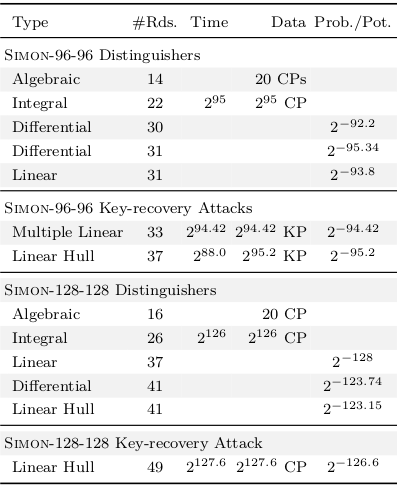Existing results of best distinguishers and best key-recovery attacks on Simon-96 in the single-key setting. Dist. = distinguisher; Prob. = probability; Pot. = linear potential.

Algebraic Cryptanalysis is unlikely to be a threat on Simon-like constructions for sufficiently many rounds. However, Raddum showed that the large number of rounds is necessary, by demonstrating that equation systems of up to 14 rounds of Simon-96-96 and up to 16 rounds of Simon-128 are efficiently solvable on an off-the-shelf laptop. Though, extensions are unknown at the moment.

Meet-in-the-Middle Attacks are successful primarily on primitives that do not use parts of the key space in sequences of several rounds. The Simon-2w-2w versions use every key bit in each sequence of two subsequent rounds, which limits the chances of meet-in-the-middle attacks drastically. Considering 3-subset meet-in-the-middle attacks, together with an initial structure and partial matching, the length of an attack is limited to that of twice the full diffusion plus four rounds plus the maximal length of an initial structure plus two rounds for a splice-and-cut part, which yiels 30 rounds as upper bound. Though, it is unlikely that such attacks cover 30 or more rounds on Simon-2w-2w.

Correlated Sequences. A recent interesting direction may be correlated sequences. Rohit and Gong’s technique requires only very few texts and could break 27 rounds of Simon-32 and Simeck-32 and thus, outperformed all previous attacks by at least 3 rounds. Though, that approach needs further investigation and has seen application only to Simon-32-64 until now.

### Implications to SimP

Clearly, the key schedule of Simon is completely linear. Therefore, the two state words that are transformed by the key schedule allow complete prediction of differences, linear and algebraic properties through a full step. Though, SimP transforms each input word through at least 52 rounds of Simon, in case of SimP-192-2 and SimP-192-4, and 68 rounds of Simon, in case of SimP-256-2 and SimP-256-4.

Related-key Differential Cryptanalysis. SimP needs an analysis of related-key differential and linear characteristics. Though, with existing methods such as the exhaustive search in or SAT solvers, such a study is difficult due to the large state size since the known tools cannot scale appropriately. There exist peer-reviewed related-key results on Simon, e.g., by Wang et al.. Though, their search restricted to related-key trails for the small variants of the Simon family, i.e., Simon-32, Simon-48, and Simon-64.
We conducted experiments using the SAT-based approach from Kölbl et al. as well as using the approach from Liu et al. to search optimal differential characteristics on SimP. Though, the related-key analysis of Simon-like constructions is computationally difficult because of the large state size. We obtained improved trails for only for up to seven rounds of Simon-96; starting from eight rounds, the best found characteristics possessed a zero key difference for up to 10 rounds, which suggests that differences in the few key words do not improve the best single-key characteristics. So, it seems that the probabilities of the existing optimal differential characteristics and linear trails for Simon-96-96 and Simon-128-128 also hold for SimP-192-1 and SimP-256-1 from there. The following table compares the probabilities of optimal single and related-key differential characteristics.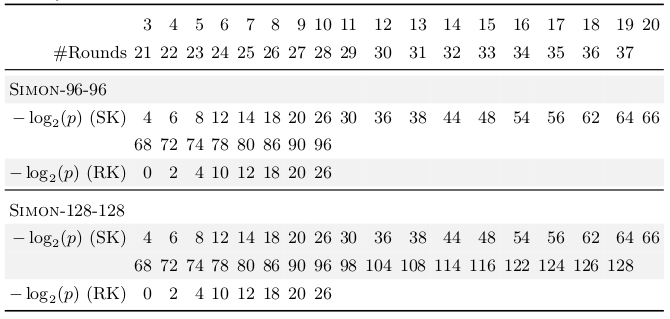Probabilities of optimal related-key differential characteristics for round-reduced variants of Simon-96-96 and Simon-128-128. p denotes the probability; SK = single-key model, RK = related-key model.

Number of Steps and Rounds of SimP. SimP benefits from the intensive cryptanalysis of Simon. The usage of the key-update function of Simon seems to not promote considerably more effective differential or linear attacks compared to the single-key results on Simon. The usage of the 2w-word key appears not exploitable neither by differentials and linear characteristics, nor by techniques that try to exploit more available state such as meet-in-the-middle attacks. The reason is in the diffusion and the relatively large number of rounds.
The number of steps and the number of rounds in our employed instantiations of SimP have been chosen very conservatively, using the number of rounds per step r s as half the number of rounds in Simon. This choice guarantees that each bit passes at least once through the full-round cipher, and therefore is expected to possess the algebraic degree of the full-round cipher. Moreover, the diffusion properties of Simon render impossible-differential, zero-correlation, or integral attacks implausible.
The design of SimP is as close to the original design of Simon as possible. Therefore, any considerable novel result in the cryptanalysis on SimP would most likely also be a higher threat on Simon-2w-2w at the same time. Thus, such results are possible, but appear unrealistic in the mid-term future. Moreover, the higher number of rounds in SimP provide an additional security margin.

## Hardware Performance

Below, we consider aspects of Oribatida when implementing it in hardware.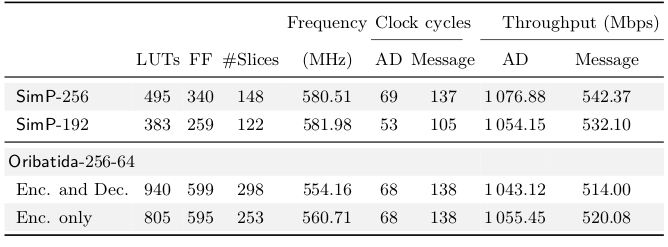Implementation results for SimP-256 and Oribatida-256-64 encryption/decryption and only encryption on Virtex 7 FPGA. LUTs = lookup tables; AD = associated data; Enc. = encryption; Dec. = decryption.

1. Oribatida Is Simple. It can be implemented efficiently with little extra cost compared to the duplex sponge. Additional costs result from the use of a module to generate the constants for the domain separation, which can be held in ROM. In modern FPGAs, this module takes only four LUTs. For domain separation, only a four-bit XOR is necessary at the input for capacity of the permutation. An additional 64-bit register to store a mask, and a 64-bit XOR to add the mask to the ciphertext is requires.

2. Oribatida Allows A Wide Spectrum of Implementations. The use of SimP as its main building block allows to directly transfer the same strategy of using different data-path sizes to Oribatida. Thus, the implementer can choose among various trade-offs between throughput, latency, area, and power consumption.

3. Side-channel Resistance. In terms of side-channel resistance, the same aspects that hold for SimP also hold for the mode Oribatida. Thus, Oribatida does not introduce additional weaknesses of side channels.
The above table lists the implementations results obtained from Xilinx Vivado 2018 optimizing for area. All results represent measurements after the place-and-route process.
In the above table, we list two columns for the number of clock cycles and throughput, the first one is for associated data processing (reduced rounds SimP) and the second for message processing (normal SimP). Our results represent the first implementation attempts of Oribatida that leaves still room for improvements as explained above.